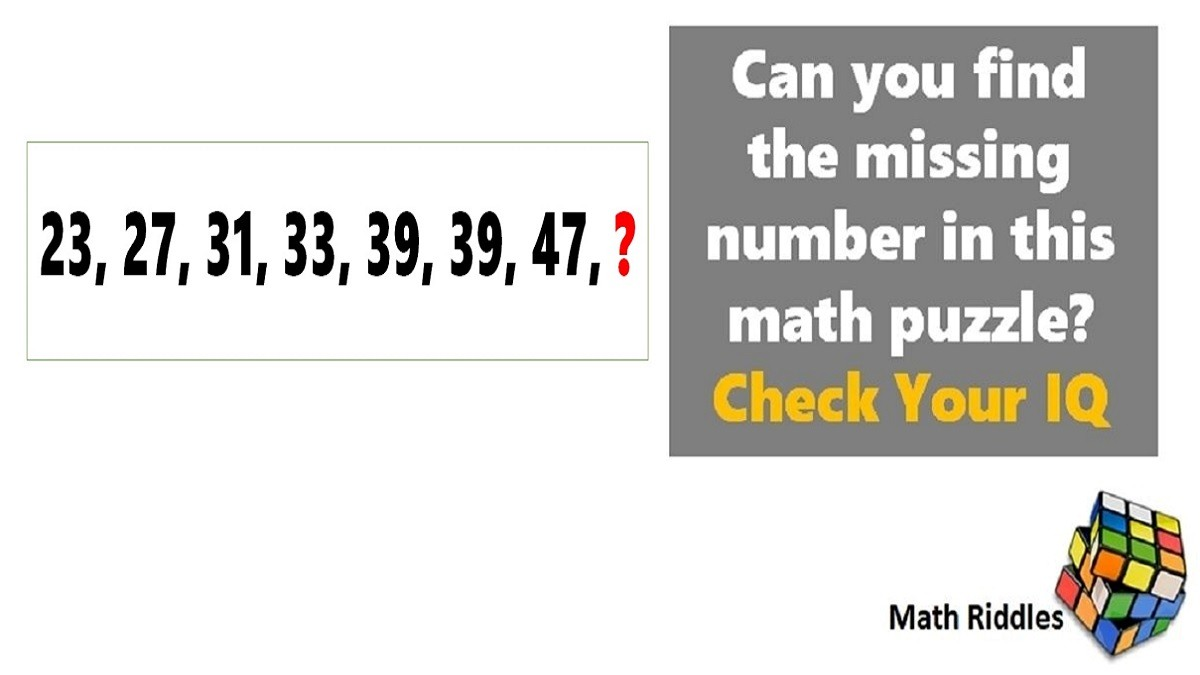# Math Riddles: Missing Numbers Series Puzzle for Genius

Math Riddles Challenge: Can you find the missing number in this math puzzle? Check Your IQMath Riddles: Missing Numbers Series Puzzle for Genius

Math Riddles are really fun way to test your maths skills. We another set of tricky math puzzle where one has to find the missing number in the place of the question mark. Today, we have two interesting math puzzles. Let us see if you can find the missing number in 30 seconds each.

Logic Puzzle #1

Logic Puzzle #2

Logic Puzzle #3

## Tell us in comments: Did you find the missing numbers correctly in 30 seconds each?

Check out more math puzzles!

Also Read: Math Riddles: Test Your IQ, Find The Missing Numbers Math Puzzles

Also Read: Math Riddles: Missing Number Math Puzzle, IQ and Aptitude Test

Also Read: Math Riddles: Can You Find the Logic in this Magic Square 3×3?

Get the latest General Knowledge and Current Affairs from all over India and world for all competitive exams.
खेलें हर किस्म के रोमांच से भरपूर गेम्स सिर्फ़ जागरण प्ले पर